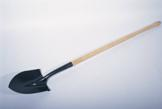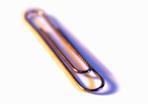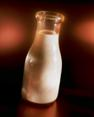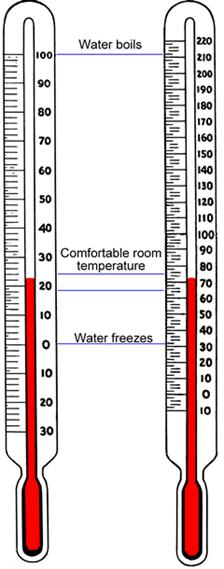## Systems and Scales of Measurement

### Learning Outcomes

• Describe the general relationship between the U.S. customary units and metric units of length, weight/mass, and volume
• Define the metric prefixes and use them to perform basic conversions among metric units
• Solve application problems using metric units
• State the freezing and boiling points of water on the Celsius and Fahrenheit temperature scales.
• Convert from one temperature scale to the other, using conversion formulas

In the United States, both the U.S. customary measurement system and the metric system are used, especially in medical, scientific, and technical fields. In most other countries, the metric system is the primary system of measurement. If you travel to other countries, you will see that road signs list distances in kilometers and milk is sold in liters. People in many countries use words like kilometer, liter, and milligram to measure the length, volume, and weight of different objects. These measurement units are part of the metric system.

Unlike the U.S. customary system of measurement, the metric system is based on 10s. For example, a liter is 10 times larger than a deciliter, and a centigram is 10 times larger than a milligram. This idea of 10 is not present in the U.S. customary systemthere are 12 inches in a foot, and 3 feet in a yard and 5,280 feet in a mile!

So, what if you have to find out how many milligrams are in a decigram? Or, what if you want to convert meters to kilometers? Understanding how the metric system works is a good start.

In this section we will discover the basic units used in the metric system, and show how to convert between them. We will also explore temperature scales. In the United States, temperatures are usually measured using the Fahrenheit scale, while most countries that use the metric system use the Celsius scale to record temperatures. Learning about the different scales, including how to convert between them will help you figure out what the weather is going to be like, no matter which country you find yourself in.

## Metric System Basics

### What Is Metric?

The metric system uses units such as meter, liter, and gram to measure length, liquid volume, and mass, just as the U.S. customary system uses feet, quarts, and ounces to measure these.

In addition to the difference in the basic units, the metric system is based on 10s, and different measures for length include kilometer, meter, decimeter, centimeter, and millimeter. Notice that the word meter is part of all of these units.

The metric system also applies the idea that units within the system get larger or smaller by a power of 10. This means that a meter is 100 times larger than a centimeter, and a kilogram is 1,000 times heavier than a gram. You will explore this idea a bit later. For now, notice how this idea of getting bigger or smaller by 10 is very different than the relationship between units in the U.S. customary system, where 3 feet equals 1 yard, and 16 ounces equals 1 pound.

### Length, Mass, and Volume

The table below shows the basic units of the metric system. Note that the names of all metric units follow from these three basic units.

 Length Mass Volume basic units meter gram liter other units you may see kilometer kilogram dekaliter centimeter centigram centiliter millimeter milligram milliliter

In the metric system, the basic unit of length is the meter. A meter is slightly larger than a yardstick, or just over three feet.

The basic metric unit of mass is the gram. A regular-sized paperclip has a mass of about 1 gram.

Among scientists, one gram is defined as the mass of water that would fill a 1-centimeter cube. You may notice that the word mass is used here instead of weight. In the sciences and technical fields, a distinction is made between weight and mass. Weight is a measure of the pull of gravity on an object. For this reason, an objects weight would be different if it was weighed on Earth or on the moon because of the difference in the gravitational forces. However, the objects mass would remain the same in both places because mass measures the amount of substance in an object. As long as you are planning on only measuring objects on Earth, you can use mass/weight fairly interchangeablybut it is worth noting that there is a difference!

Finally, the basic metric unit of volume is the liter. A liter is slightly larger than a quart.The handle of a shovel is about 1 meter. A paperclip weighs about 1 gram. A medium-sized container of milk is about 1 liter.

Though it is rarely necessary to convert between the customary and metric systems, sometimes it helps to have a mental image of how large or small some units are. The table below shows the relationship between some common units in both systems.

 Common Measurements in Customary and Metric Systems Length 1 centimeter is a little less than half an inch. 1.6 kilometers is about 1 mile. 1 meter is about 3 inches longer than 1 yard. Mass 1 kilogram is a little more than 2 pounds. 28 grams is about the same as 1 ounce. Volume 1 liter is a little more than 1 quart. 4 liters is a little more than 1 gallon.

### Prefixes in the Metric System

The metric system is a base 10 system. This means that each successive unit is 10 times larger than the previous one.

The names of metric units are formed by adding a prefix to the basic unit of measurement. To tell how large or small a unit is, you look at the prefix. To tell whether the unit is measuring length, mass, or volume, you look at the base.

 Prefixes in the Metric System kilo- hecto- deka- meter gram liter deci- centi- milli- 1,000 times larger than base unit 100 times larger than base unit 10 times larger than base unit base units 10 times smaller than base unit 100 times smaller than base unit 1,000 times smaller than base unit

Using this table as a reference, you can see the following:

• A kilogram is 1,000 times larger than one gram (so 1 kilogram = 1,000 grams).
• A centimeter is 100 times smaller than one meter (so 1 meter = 100 centimeters).
• A dekaliter is 10 times larger than one liter (so 1 dekaliter = 10 liters).

Here is a similar table that just shows the metric units of measurement for mass, along with their size relative to 1 gram (the base unit). The common abbreviations for these metric units have been included as well.

 Measuring Mass in the Metric System kilogram (kg) hectogram (hg) dekagram (dag) gram (g) decigram (dg) centigram (cg) milligram (mg) 1,000 grams 100 grams 10 grams gram 0.1 gram 0.01 gram 0.001 gram

Since the prefixes remain constant through the metric system, you could create similar charts for length and volume. The prefixes have the same meanings whether they are attached to the units of length (meter), mass (gram), or volume (liter).

### Try It

Which of the following sets of three units are all metric measurements of length?

A) inch, foot, yard

B) kilometer, centimeter, millimeter

C) kilogram, gram, centigram

D) kilometer, foot, decimeter

### Converting Units Up and Down the Metric Scale

Converting between metric units of measure requires knowledge of the metric prefixes and an understanding of the decimal systemthats about it.

For instance, you can figure out how many centigrams are in one dekagram by using the table above. One dekagram is larger than one centigram, so you expect that one dekagram will equal many centigrams.

In the table, each unit is 10 times larger than the one to its immediate right. This means that 1 dekagram = 10 grams; 10 grams = 100 decigrams; and 100 decigrams = 1,000 centigrams. So, 1 dekagram = 1,000 centigrams.

### Example

How many milligrams are in one decigram?

### Try It

Convert 3,085 milligrams to grams.

### Example

Convert 1 centimeter to kilometers.

### Try It

Once you begin to understand the metric system, you can use a shortcut to convert among different metric units. The size of metric units increases tenfold as you go up the metric scale. The decimal system works the same way: a tenth is 10 times larger than a hundredth; a hundredth is 10 times larger than a thousandth, etc. By applying what you know about decimals to the metric system, converting among units is as simple as moving decimal points.

Here is the first problem from above: How many milligrams are in one decigram? You can recreate the order of the metric units as shown below:

$\displaystyle kg\quad hg\quad dag\quad g\quad d\underbrace{g\quad c}_{1}\underbrace{g\quad m}_{2}g$

This question asks you to start with 1 decigram and convert that to milligrams. As shown above, milligrams is two places to the right of decigrams. You can just move the decimal point two places to the right to convert decigrams to milligrams: $\displaystyle 1\ dg=1\underbrace{0}_{1}\underbrace{0}_{2}.\ mg$.

The same method works when you are converting from a smaller to a larger unit, as in the problem: Convert 1 centimeter to kilometers.

$\displaystyle k\underbrace{m\quad h}_{5}\underbrace{m\quad d}_{4}\underbrace{am\quad }_{3}\underbrace{m\quad d}_{2}\underbrace{m\quad c}_{1}m\quad mm$

Note that instead of moving to the right, you are now moving to the leftso the decimal point must do the same:

$\displaystyle 1\ cm=0.\underbrace{0}_{5}\underbrace{0}_{4}\underbrace{0}_{3}\underbrace{0}_{2}\underbrace{1}_{1}\ km$.

### Try It

How many milliliters are in 1 liter?

### Factor Label Method

There is yet another method that you can use to convert metric measurementsthe factor label method. You used this method when you were converting measurement units within the U.S. customary system.

The factor label method works the same in the metric system; it relies on the use of unit fractions and the cancelling of intermediate units. The table below shows some of the unit equivalents and unit fractions for length in the metric system. (You should notice that all of the unit fractions contain a factor of 10. Remember that the metric system is based on the notion that each unit is 10 times larger than the one that came before it.)

Also, notice that two new prefixes have been added here: mega- (which is very big) and micro- (which is very small).

 Unit Equivalents Conversion Factors 1 meter = 1,000,000 micrometers $\displaystyle \frac{1\ m}{1,000,000\ \mu m}$ $\displaystyle \frac{1,000,000\ \mu m}{1\ m}$ 1 meter = 1,000 millimeters $\displaystyle \frac{1\ m}{1,000\ mm}$ $\displaystyle \frac{1,000\ mm}{1\ m}$ 1 meter = 100 centimeters $\displaystyle \frac{1\ m}{100\ cm}$ $\displaystyle \frac{100\ cm}{1\ m}$ 1 meter = 10 decimeters $\displaystyle \frac{1\ m}{10\ dm}$ $\displaystyle \frac{10\ dm}{1\ m}$ 1 dekameter = 10 meters $\displaystyle \frac{1\ dam}{10\ m}$ $\displaystyle \frac{10\ m}{1\ dam}$ 1 hectometer = 100 meters $\displaystyle \frac{1\ hm}{100\ m}$ $\displaystyle \frac{100\ m}{1\ hm}$ 1 kilometer = 1,000 meters $\displaystyle \frac{1\ km}{1,000\ m}$ $\displaystyle \frac{1,000\ m}{1\ km}$ 1 megameter = 1,000,000 meters $\displaystyle \frac{1\ Mm}{1,000,000\ m}$ $\displaystyle \frac{1,000,000\ m}{1\ Mm}$

When applying the factor label method in the metric system, be sure to check that you are not skipping over any intermediate units of measurement!

### Example

Convert 7,225 centimeters to meters.

### Try It

Convert 32.5 kilometers to meters.

Now that you have seen how to convert among metric measurements in multiple ways, let’s revisit the problem posed earlier.

### Example

If you have a prescription for 5,000 mg of medicine, and upon getting it filled, the dosage reads 5g of medicine, did the pharmacist make a mistake?

## Applications of Metric Conversions

Learning how to solve real-world problems using metric conversions is as important as learning how to do the conversions themselves. Mathematicians, scientists, nurses, and even athletes are often confronted with situations where they are presented with information using metric measurements, and must then make informed decisions based on that data.

TIP: To solve these problems effectively, you need to understand the context of a problem, perform conversions, and then check the reasonableness of your answer. Do all three of these steps and you will succeed in whatever measurement system you find yourself using.

### Understanding Context and Performing Conversions

The first step in solving any real-world problem is to understand its context. This will help you figure out what kinds of solutions are reasonable (and the problem itself may give you clues about what types of conversions are necessary). Here is an example.

### Example

Marcus bought at 2 meter board, and cut off a piece 1 meter and 35 cm long. How much board is left?

An example with a different context, but still requiring conversions, is shown below.

### Example

A faucet drips 10 ml every minute. How much water will be wasted in a week?

This problem asked for the difference between two quantities. The easiest way to find this is to convert one quantity so that both quantities are measured in the same unit, and then subtract one from the other.

### Try It

A bread recipe calls for 600 g of flour. How many kilograms of flour would you need to make 5 loaves?

Sometimes it is a good idea to check your conversions using a second method. This usually helps you catch any errors that you may make, such as using the wrong unit fractions or moving the decimal point the wrong way.

### Example

A bottle contains 1.5 liters of a beverage. How many 250 mL servings can be made from that bottle?

Understanding the context of real-life application problems is important. Look for words within the problem that help you identify what operations are needed, and then apply the correct unit conversions. Checking your final answer by using another conversion method (such as the move the decimal method, if you have used the factor label method to solve the problem) can cut down on errors in your calculations.

### Summary

The metric system is an alternative system of measurement used in most countries, as well as in the United States. The metric system is based on joining one of a series of prefixes, including kilo-, hecto-, deka-, deci-, centi-, and milli-, with a base unit of measurement, such as meter, liter, or gram. Units in the metric system are all related by a power of 10, which means that each successive unit is 10 times larger than the previous one.

This makes converting one metric measurement to another a straightforward process, and is often as simple as moving a decimal point. It is always important, though, to consider the direction of the conversion. If you are converting a smaller unit to a larger unit, then the decimal point has to move to the left (making your number smaller); if you are converting a larger unit to a smaller unit, then the decimal point has to move to the right (making your number larger).

The factor label method can also be applied to conversions within the metric system. To use the factor label method, you multiply the original measurement by unit fractions; this allows you to represent the original measurement in a different measurement unit.

## Temperature Scales

Turn on the television any morning and you will see meteorologists talking about the days weather forecast. In addition to telling you what the weather conditions will be like (sunny, cloudy, rainy, muggy), they also tell you the days forecast for high and low temperatures. A hot summer day may reach 100° in Philadelphia, while a cool spring day may have a low of 40° in Seattle.

If you have been to other countries, though, you may notice that meteorologists measure heat and cold differently outside of the United States. For example, a TV weatherman in San Diego may forecast a high of 89°, but a similar forecaster in Tijuana, Mexico, which is only 20 miles south, may look at the same weather pattern and say that the day’s high temperature is going to be 32°. Whats going on here? The difference is that the two countries use different temperature scales.

### Measuring Temperature on Two Scales

Fahrenheit and Celsius are two different scales for measuring temperature.

 A thermometer measuring a temperature of 22° Celsius is shown here. On the Celsius scale, water freezes at 0° and boils at 100°. If the United States were to adopt the Celsius scale, forecast temperatures would rarely go below -30° or above 45°. (A temperature of -18° may be forecast for a cold winter day in Michigan, while a temperature of 43° may be predicted for a hot summer day in Arizona.) Most office buildings maintain an indoor temperature between 18°C and 24°C to keep employees comfortable.A thermometer measuring a temperature of 72° Fahrenheit is shown here. On the Fahrenheit scale, water freezes at 32° and boils at 212°. In the United States, forecast temperatures measured in Fahrenheit rarely go below -20° or above 120°. (A temperature of 0° may be forecast for a cold winter day in Michigan, while a temperature of 110° may be predicted for a hot summer day in Arizona.) Most office buildings maintain an indoor temperature between 65°F and 75°F to keep employees comfortable. Celsius Fahrenheit

### Try It

A cook puts a thermometer into a pot of water to see how hot it is. The thermometer reads 132°, but the water is not boiling yet. Which temperature scale is the thermometer measuring?

### Converting Between the Scales

By looking at the two thermometers shown, you can make some general comparisons between the scales. For example, many people tend to be comfortable in outdoor temperatures between 50°F and 80°F (or between 10°C and 25°C). If a meteorologist predicts an average temperature of 0°C (or 32°F), then it is a safe bet that you will need a winter jacket.

Sometimes, it is necessary to convert a Celsius measurement to its exact Fahrenheit measurement or vice versa. For example, what if you want to know the temperature of your child in Fahrenheit, and the only thermometer you have measures temperature in Celsius measurement? Converting temperature between the systems is a straightforward process as long as you use the formulas provided below.

### Temperature Conversion Formulas

To convert a Fahrenheit measurement to a Celsius measurement, use this formula.

$C=\frac{5}{9}(F-32)$

To convert a Celsius measurement to a Fahrenheit measurement, use this formula.

$F=\frac{9}{5}C+32$

How were these formulas developed? They came from comparing the two scales. Since the freezing point is 0° in the Celsius scale and 32° on the Fahrenheit scale, we subtract 32 when converting from Fahrenheit to Celsius, and add 32 when converting from Celsius to Fahrenheit.

There is a reason for the fractions $\frac{5}{9}$ and $\frac{9}{5}$, also. There are 100 degrees between the freezing (0°) and boiling points (100°) of water on the Celsius scale and 180 degrees between the similar points (32° and 212°) on the Fahrenheit scale. Writing these two scales as a ratio, $\frac{F{}^\circ }{C{}^\circ }$, gives $\frac{180{}^\circ }{100{}^\circ }=\frac{180{}^\circ \div 20}{100{}^\circ \div 20}=\frac{9}{5}$. If you flip the ratio to be $\frac{\text{C}{}^\circ }{\text{F}{}^\circ }$, you get $\frac{100{}^\circ }{180{}^\circ }=\frac{100{}^\circ \div 20}{180{}^\circ \div 20}=\frac{5}{9}$. Notice how these fractions are used in the conversion formulas.

The example below illustrates the conversion of Celsius temperature to Fahrenheit temperature, using the boiling point of water, which is 100° C.

### Example

The boiling point of water is 100°C. What temperature does water boil at in the Fahrenheit scale?

A Celsius temperature is given. To convert it to the Fahrenheit scale, use the formula at the left.

$F=\frac{9}{5}C+32$

Substitute 100 for C and multiply.

$F=\frac{9}{5}(100)+32$

$F=\frac{900}{5}+32$

Simplify $\frac{900}{5}$ by dividing numerator and denominator by 5.

$F=\frac{900\div 5}{5\div 5}+32$

$F=\frac{180}{1}+32$

Add $180+32$.

$F=212$

The boiling point of water is 212°F.

### Example

Water freezes at 32°F. On the Celsius scale, what temperature is this?

### TRY IT NOW

The two previous problems used the conversion formulas to verify some temperature conversions that were discussed earlierthe boiling and freezing points of water. The next example shows how these formulas can be used to solve a real-world problem using different temperature scales.

### Example

Two scientists are doing an experiment designed to identify the boiling point of an unknown liquid. One scientist gets a result of 120°C; the other gets a result of 250°F. Which temperature is higher and by how much?

You could have converted 250°F to °C instead, and then found the difference in the two measurements. (Had you done it this way, you would have found that $250^{\circ}\text{F}=121.1^{\circ}\text{C}$, and that 121.1°C is 1.1°C higher than 120°C.) Whichever way you choose, it is important to compare the temperature measurements within the same scale, and to apply the conversion formulas accurately.

### Try It

Tatiana is researching vacation destinations, and she sees that the average summer temperature in Barcelona, Spain is around 26°C. What is the average temperature in degrees Fahrenheit?

### Summary

Temperature is often measured in one of two scales: the Celsius scale and the Fahrenheit scale. A Celsius thermometer will measure the boiling point of water at 100° and its freezing point at 0°; a Fahrenheit thermometer will measure the same events at 212° for the boiling point of water and 32° as its freezing point. You can use conversion formulas to convert a measurement made in one scale to the other scale.